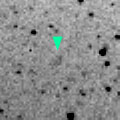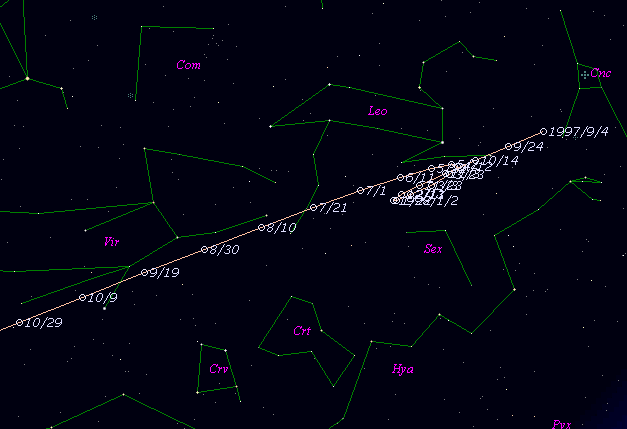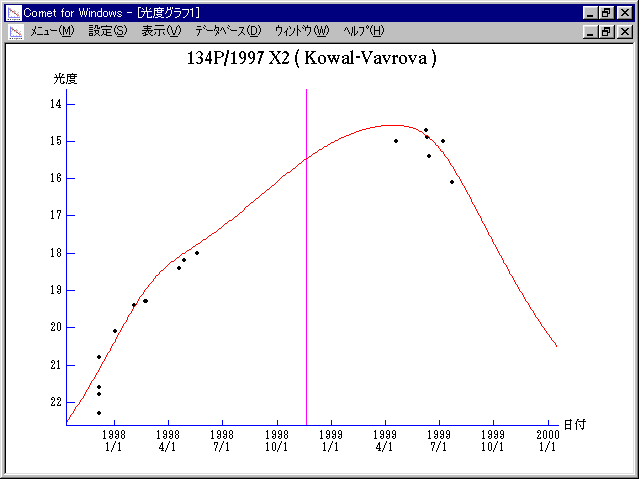# \$B%3%o%k(B-\$B%t%!!<%t%m%t%!!

134P/Kowal-Vavrova (1998)###\$B%W%m%U%#!<%k(B

 \$BId9f(B 134P/1997 X2 \$B8!=PF|(B 1997\$BG/(B12\$B7n(B5\$BF|(B \$B8!=P8wEY(B 21.8\$BEy(B \$B8!=P J. V. Scotti (Kitt Peak)

###\$B###\$B50F;MWAG(B

```   The following improved orbital elements by Kenji Muraoka, are
from 52 observations 1983 May 8 to 1998 June 18, perturbations
by 9 Plantes, Moon and 5 minor planets were taken into account.
The mean residual is +/- 0.63 arc seconds.

Epoch  =  1998 Nov.  3.0  TT       JDT = 2451120.5
T  =  1998 Nov. 18.85374       +/- 0.00132 (m.e.) TT
Peri. =   18.71177                +/- 0.00055
Node  =  202.28607                +/- 0.00045   (2000.0)
Incl. =    4.34521                +/- 0.00003
q  =    2.5753243              +/- 0.0000091 AU
e  =    0.5871919              +/- 0.0000013
a  =    6.2385515              +/- 0.0000048 AU
n  =    0.06325261             +/- 0.00000007
P  =   15.582                  +/- 0.0000179  years
```

###\$B@1?^(B###\$B8wEYJQ2=(B

```        m1 = 0 + 5 log\$B&\$(B + 30 log r(t - 80)
```##### \$B50F;MWAG\$OB<2,7r<#;a\$N7W;;\$K\$h\$k\$b\$N\$G\$9!#(B \$B@1?^\$O(B StellaNavigator Ver.2.0 for Windows (\$B%"%9%H%m%"!<%D(B \$BJTCx(B / \$B%"%9%-!<=PHG6I4)(B) \$B\$G:n@.\$7\$?\$b\$N\$G\$9!#(B \$B8wEY%0%i%U\$O(BComet for Windows\$B\$G:n@.\$7\$?\$b\$N\$G\$9!#(B